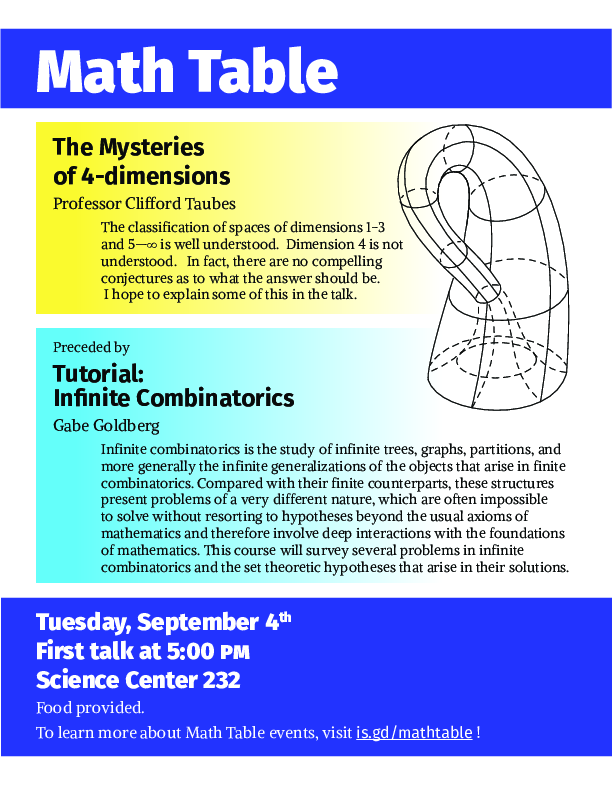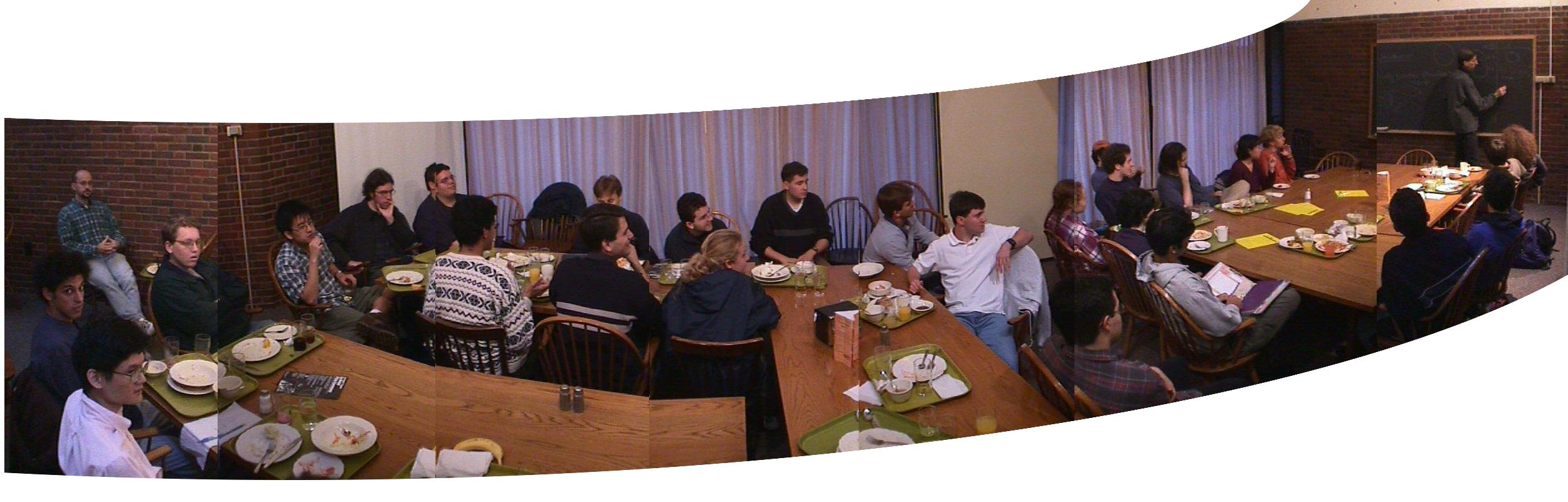Department of Mathematics FAS Harvard University One Oxford Street Cambridge MA 02138 USA Tel: (617) 495-2171 Fax: (617) 495-5132

### Math table September 4, 2018The first Math Table will be held in the Science Center on Tuesday, September 4, on the second floor in room 232. There will be an overview of the fall tutorial and a talk given by Prof. Cliff Taubes. The first talk will begin at 5:00 p.m. Gabe Goldberg, Harvard University, will be giving an overview of the fall 2018 tutorial titled:

#### Infinite Combinatorics

Description: Infinite combinatorics is the study of infinite trees, graphs, partitions, and more generally the infinite generalizations of the objects that arise in finite combinatorics. Compared with their finite counterparts, these structures present problems of a very different nature, which are often impossible to solve without resorting to hypotheses beyond the usual axioms of mathematics and therefore involve deep interactions with the foundations of mathematics. This course will survey several problems in infinite combinatorics and the set theoretic hypotheses that arise in their solutions. Possible topics: are Erdös's partition calculus, the non-stationary ideal, ultrafilters, Suslin's problem, the Singular Cardinals Problem, large cardinal axioms, the Continuum Hypothesis, Martin's Axiom, the Axiom of Determinacy.

Prof. Cliff Taubes, Harvard University, will give a talk titled:

#### The mysteries of 4-dimensions.

Abstract: The classification of spaces of dimensions 1-3 and 5-# is well understood. Dimension 4 is not understood. In fact, there are no compelling conjectures as to what the answer should be. I hope to explain some of this in the talk. Prof. Taubes will be available after the talks to sign students up for the fall tutorial.Caption: Cliff Taubes giving a mathtable talk in 2000